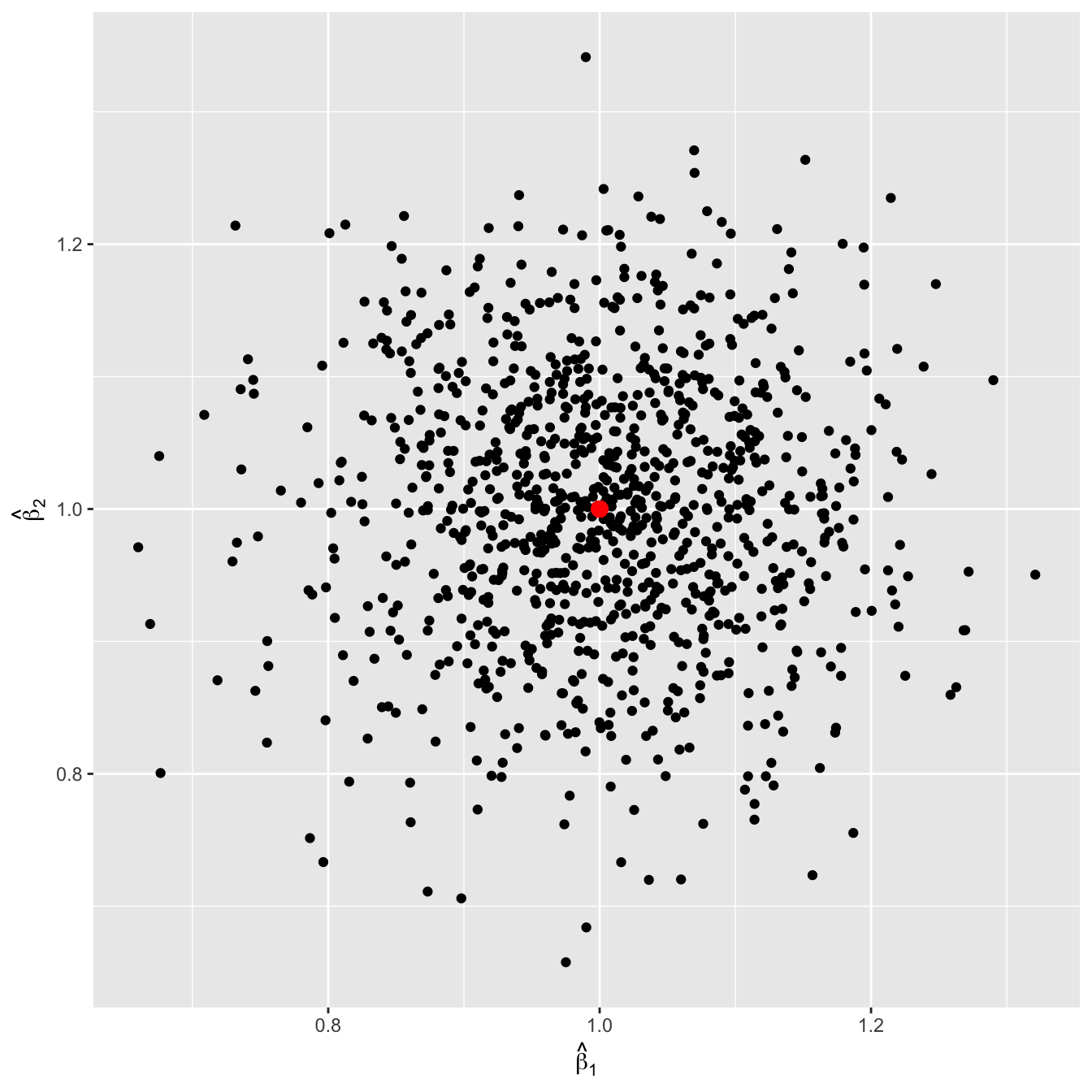# Introduction

While there are many resources out there to describe the issues arising with multicollinearity in independent variables, there’s a visualization for the problem that I came across in a book once and haven’t found replicated online. This replicates the visualization.

# Set-up

Let $$Y$$ be a response and $$X_1$$ and $$X_2$$ be the predictors, such that

$Y_i = \beta_0 + \beta_1X_{1i} + \beta_2X_{2i} + \epsilon_i$

for individual $$i$$.

For simplicity, let’s say that

$\beta_0 = 0,\\ \beta_1 = 1,\\ \beta_2 = 1.$

I carry out a simulation by generating 1,000 simulations with a given correlation between predictors and obtain their coefficients.

reps <- 1000
n <- 100
save <- matrix(nrow = reps, ncol = 3)

for (i in 1:reps) {
x1 <- rnorm(n)
x2 <- rnorm(n)
y <- x1 + x2 + rnorm(n)

mod <- lm(y ~ x1 + x2)
save[i,] <- c(coef(mod)[-1], cor(x1, x2))
}

The line x2 <- rnorm(n) gets replaced with x2 <- x1 + rnorm(n, sd = _), where the _ is replaced with difference values to induce more correlation between x1 and x2.

Following these simulations, the coefficients are plotted against each out.

# Simulation

## No Collinearity

The average correlation between $$X_1$$ and $$X_2$$ is 0.007.The red dot represents the true coefficients. We see no relationship between the estimated coefficients, and each are well centered around the truth.

## Moderate Collinearity of .5

The average correlation between $$X_1$$ and $$X_2$$ is 0.556.